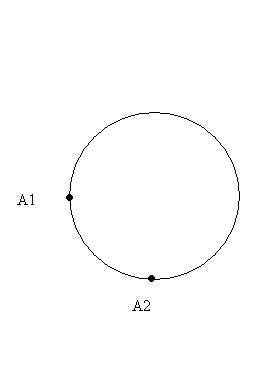# Physics: Lenz's Law/Induction

## Recommended Posts

I have circular wire loop (radius 10 cm) that exists in the presence of a growing magnetic field. Thus, an EMF of 5 V is induced in the wire. We are given that the wire resistance is a constant 2 ohms/cm. Two arbitrary points are chosen on the wire, A1 and A2. These points divide the wire into two arcs, L1 and L2, where L1 = 3L2. Now, the question asks: What is the electric potential from A1 to A2 along the longer arc? And along the shorter arc?

My book explains clearly that "Electric potential does not have meaning for electric fields produced by induction".

So...is this a trick question (which it might very well be, my professor has done so in the past)? Thanks.##### Share on other sites

It looks like it very well could be. Since the main information you know is the fact that the loop has an EMF of 5V, which means the potential difference is 5V and the potential difference is $V(b) - V(a) = - \int_a^b \mathbf{E} \cdot d\mathbf{l}$

Edit: Or you could just say $\varepsilon = \oint \mathbf{E} \cdot d\mathbf{l}$, where $\varepsilon$ is the EMF. So the electric potential is described fully as $V® = - \int_o^r \mathbf{E} \cdot d\mathbf{l}$, where o is the origin point of choosing.

Edited by darkenlighten
##### Share on other sites

I'd say that since the wire has a given resistance, it'll have a current running through the loop. You can use that resistance to find the potential drop over a portion of the loop.

On the other hand, I'll bet it doesn't quite work that way, since the field is symmetric. How can you get a potential difference when the ring and field are completely symmetric?

##### Share on other sites

It looks like it very well could be. Since the main information you know is the fact that the loop has an EMF of 5V, which means the potential difference is 5V and the potential difference is $V(b) - V(a) = - \int_a^b \mathbf{E} \cdot d\mathbf{l}$

Edit: Or you could just say $\varepsilon = \oint \mathbf{E} \cdot d\mathbf{l}$

Now take the next step of making a and b points adjacent to A1 (or A2), just to either side. If the point were a battery, it would make sense, but not for induction. The potential does not jump from 0 to 5V at any one point.

##### Share on other sites

And this does depend on whether or not they are asking for the electric potential or the potential difference, since those are two different things.

It seems like it wants the potential difference, considering it is between two points, even though the questions states electric potential. And also due to the nature of this problem, if it does have an answer, which I believe it should, the two different arcs will have the same potential difference. I would like to wait for the poster's response before I go further.

## Create an account

Register a new account